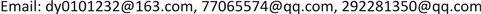1海南电网有限责任公司电力科学研究院，海南 海口

2海南省电网理化分析重点实验室，海南 海口1. 引言

2. 大规模电力系统动态频率响应特性2.1. 多机电力系统动态频率过程

P i ≅ ∑ j = 1 j ≠ i n E i E j B i j sin δ i j + E i V k B i k sin δ i k (1)

P k ≅ ∑ j = 1 n V k E j B k j sin δ k j (2)

p i Δ = ∑ j = 1 j ≠ i n ( E i E j E i j cos δ i j 0 ) δ i j Δ + ( E i V k B i k cos δ i k 0 ) δ i k Δ (3)

P k Δ = ∑ j = 1 n ( V k E j B k j cos δ k j 0 ) δ k j Δ (4)

P S i j = V i V j B i j cos δ i j 0 (5)

P i Δ = ∑ j = 1 j ≠ i n P S i j δ i j Δ + P S i k δ i k Δ (6)

P k Δ = ∑ j = 1 n P S k j δ k j Δ (7)

P i Δ ( 0 + ) = − P S i k δ i k Δ ( 0 + ) (8)

P k Δ ( 0 + ) = ∑ j = 1 n P S k j δ k j Δ ( 0 + ) (9)

P L Δ ( 0 + ) = − P k Δ ( 0 + ) = − ∑ i = 1 n P i Δ ( 0 + ) (10)

P i Δ ( 0 + ) = P S i k ∑ j = 1 n P S j k P L Δ ( 0 + ) , i = 1 , 2 , ⋯ , n (11)

2 H i ω N d Δ ω i d t = P S i k ∑ j = 1 n P S j k P L Δ ( 0 + ) , i = 1 , 2 , ⋯ , n (12)

d f i d t = − f 0 2 H i P S i k ∑ j = 1 n P S j k P L Δ ( 0 + ) , i = 1 , 2 , ⋯ , n (13)

2.2. 动态频率的分区响应特性

3. 节点间动态频率关联性分析3.1. 基于结构保持模型的状态空间模型

P L i = P L i 0 + D i δ i · , i = n + 1 , ⋯ , n 0 (14)

2 H i δ i · · + D i δ i · + f ( δ ) = P M i 0 − P L i , i = 1 , 2 , ⋯ , n 0 (15)

H i = 0 , i = n + 1 , 2 , ⋯ , n 0

D i > 0 , i = 1 , 2 , ⋯ , n 0

P L i = 0 , i = 1 , 2 , ⋯ , n

P M i = 0 , i = n + 1 , 2 , ⋯ , n 0

f ( δ ) = ∑ j = 1 j ≠ i n 0 B i j sin ( δ i − δ j )

D i δ i · + f ( δ ) = − P L i , i = n + 1 , ⋯ , n 0 (16)

[ Δ δ · ] = [ − D L F ( δ 0 ) ] [ Δ δ ] = [ A ] ⋅ [ Δ δ ] (17)

F i j ( δ ) = ∂ f i ( δ ) / ∂ δ j = { − V i V j B i j cos δ i j , i ≠ j V i ∑ j = 1 j ≠ i n V j B i j cos δ i j , i = j

[ Δ ω ] = [ A ] ⋅ [ Δ δ ] (18)

3.2. 基于RGA的动态频率耦合系数

λ i j = ∂ y i / ∂ u j | Δ u k = 0 , k ≠ j ∂ y i / ∂ u j | Δ y k = 0 , k ≠ i = g i j [ G ] j i − 1 (19)

Λ ( G ) = { λ i j | i , j = 1 , 2 , ⋯ , n } = G ⊗ G − T (20)

R ( A ) = A ⊗ A − T (21)

Γ ( A ) = 1 2 [ d i a g { R ( A ) } − 1 R ( A ) + R ( A ) d i a g { R ( A ) } − 1 ] (22)

3.3. 基于DRGA的动态分区方法

4. 仿真与分析

Results of measure of interactio

{59, 61}0.0096{47, 49}0.0296{47,49}0.0005{80, 96}0.0350{76,77}0.0023{80, 96}0.0357
{54, 59}0.0131{38, 65}0.0308{76,77}0.0023{24, 70}0.0376{70,75}0.0045{24, 70}0.0362
{59, 60}0.0142{92, 100}0.0327{70,75}0.0052{80, 99}0.0455{47,49}0.0096{80, 99}0.0456
{19, 34}0.0155{80, 99}0.0335{19,34}0.0143{34, 43}0.0486{45,49}0.0147{77, 82}0.0497
{70, 75}0.0173{24, 70}0.0341{59,61}0.0156{77, 82}0.0495{19,34}0.0152{34, 43}0.0521
{70, 74}0.0184{49, 54}0.0387{54,59}0.0158{17, 31}0.0539{38,65}0.0158{17, 31}0.0547
{55, 59}0.0187{62, 67}0.0447{45,49}0.0186{62, 67}0.0554{54,59}0.0164{62, 67}0.0572
{79, 80}0.0219{64, 61}0.0479{59,60}0.0204{71, 72}0.0606{59,61}0.0166{71, 72}0.0618
{37, 40}0.0231{77, 80}0.0491{37, 40}0.0207{46, 48}0.0627{59,60}0.0215{46, 48}0.0643
{56, 59}0.0241{17, 31}0.0544{55, 59}0.0216{32, 113}0.0656{37,40}0.0220{32, 113}0.0666
{45, 49}0.0248{34, 43}0.0553{70, 74}0.0280{92, 94}0.0681{55,59}0.0223{92, 94}0.0681
{80, 96}0.0253{75, 77}0.0593{38, 65}0.0282{37, 39}0.0687{70,74}0.0288{79, 80}0.0709
{77, 82}0.0277{46, 48}0.0598{56, 59}0.0284{79, 80}0.0711{56,59}0.0293{37, 39}0.0716
{49, 69}0.0284{71, 72}0.0639{75, 77}0.0299{33, 37}0.0721{75,77}0.0301{100, 106}0.0743
{59, 61}0.0096{92, 94}0.0661{92,100}0.0343{100, 106}0.0746{92,100}0.0341{66, 67}0.0357

The critical measure of interactio

2分区0.07350.06900.0720
3分区0.09400.10100.1025

The load flow between different are

CB10

CB20

CB200

Case 1：运行方式1下区域A内发生300 MW有功缺额扰动；

Case 2：运行方式3下区域B内发生150 MW有功缺额扰动；

Space-time distribution matrix of Case

1A−0.95860.131.31884.60
12A−0.93750.141.31954.61
72A−0.76880.111.30184.54
30A−0.97390.131.31774.55
37A−0.73310.171.29914.55
38A−0.74820.181.29914.54
113A−0.96090.141.31834.54
43B−0.40770.281.29385.52
58B−0.27690.301.28645.59
61B−0.28420.301.28845.65
65B−0.35900.311.27935.61
71B−0.35150.281.28435.52
77B−0.31150.321.27645.59
99B−0.29480.321.28985.48
100C−0.15420.371.29495.09
88C−0.15550.381.29425.06
93C−0.17340.371.29195.07
96C−0.13230.381.28535.07
101C−0.15570.371.29425.08
112C−0.10050.391.31835.10

Case 2的动态频率响应如图6所示，其时空分布特征矩阵计算结果如表5。Case 2的计算结果同样表明，受扰后的系统动态频率所表现出的分区响应特性与本文分割结果相同，限于篇幅，本文在此不再做详细分析。

Space-time distribution matrix of Case

1A−0.10950.760.510694.36
12A−0.10790.760.511484.37
72A−0.15580.720.50504.41
30A−0.13020.740.50564.41
37A−0.14290.740.50414.39

Continued

5. 结论

1) 电力系统动态频率响应具有明显的分区响应特征；

2) 电力系统动态频率的分区响应由系统结构及参数等固有特性决定，与运行方式及扰动大小和位置无关；

3) 本文提出的动态分区方法物理概念清晰，实现简单，所得分区内各节点的动态频率响应的时空分布特征相近；

4) 动态频率分区响应特性分析结果对电力系统动态频率紧急控制方案设计具有重要的指导作用。